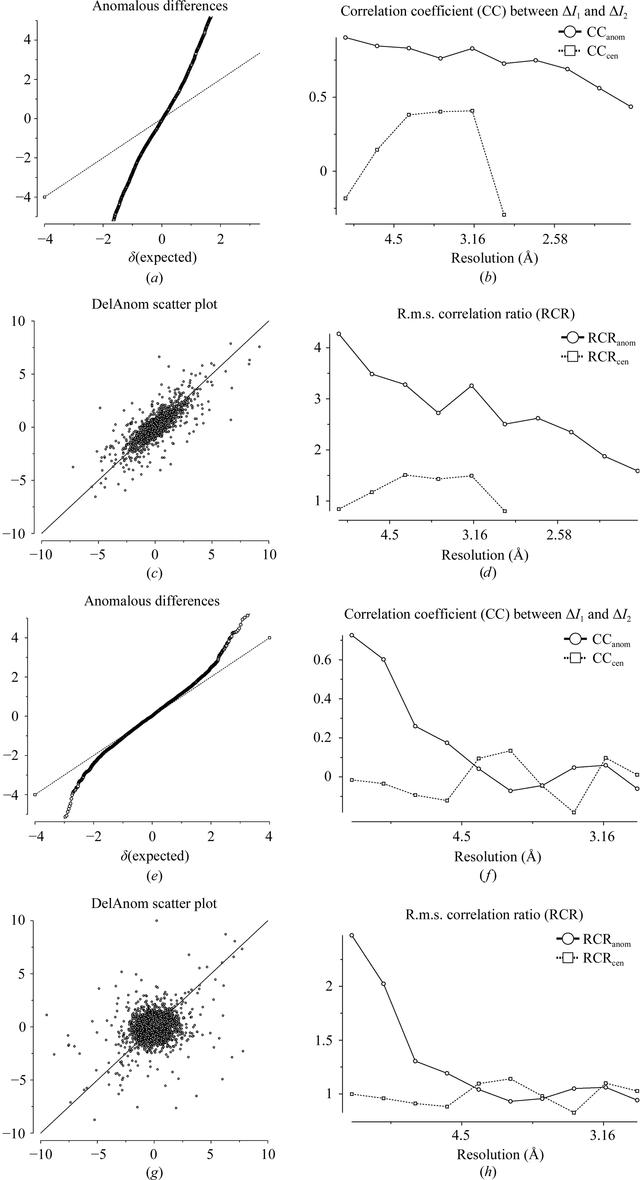disable zoom     view article Figure 5 Detection of anomalous signal. (a–d) An example with a very strong anomalous signal, shown by (a) a large slope of the normal probability plot of ΔI/σ(ΔI) values, (b) a large correlation coefficient between two ΔI estimates from random half-data sets, (c) a scatter plot relating two half-data-set values of ΔI/σ(ΔI) and (d) the r.m.s. correlation ratio derived from the scatter plot. (e–h) The same plots for an example with a weak but still useful anomalous signal.BIOLOGICALCRYSTALLOGRAPHY
ISSN: 1399-0047
Volume 67| Part 4| March 2011| Pages 282-292